Test: Magnetically Coupled Circuits

# Test: Magnetically Coupled Circuits

Test Description

## 10 Questions MCQ Test Topicwise Question Bank for Electronics Engineering | Test: Magnetically Coupled Circuits

Test: Magnetically Coupled Circuits for Electrical Engineering (EE) 2022 is part of Topicwise Question Bank for Electronics Engineering preparation. The Test: Magnetically Coupled Circuits questions and answers have been prepared according to the Electrical Engineering (EE) exam syllabus.The Test: Magnetically Coupled Circuits MCQs are made for Electrical Engineering (EE) 2022 Exam. Find important definitions, questions, notes, meanings, examples, exercises, MCQs and online tests for Test: Magnetically Coupled Circuits below.
Solutions of Test: Magnetically Coupled Circuits questions in English are available as part of our Topicwise Question Bank for Electronics Engineering for Electrical Engineering (EE) & Test: Magnetically Coupled Circuits solutions in Hindi for Topicwise Question Bank for Electronics Engineering course. Download more important topics, notes, lectures and mock test series for Electrical Engineering (EE) Exam by signing up for free. Attempt Test: Magnetically Coupled Circuits | 10 questions in 30 minutes | Mock test for Electrical Engineering (EE) preparation | Free important questions MCQ to study Topicwise Question Bank for Electronics Engineering for Electrical Engineering (EE) Exam | Download free PDF with solutions
 1 Crore+ students have signed up on EduRev. Have you?
Test: Magnetically Coupled Circuits - Question 1

### For an ideal transformer

Test: Magnetically Coupled Circuits - Question 2

### Two inductors of values L1 and L2 are coupled by a mutual inductance M. By interconnection of the two elements, one can have a minimum inductance of

Detailed Solution for Test: Magnetically Coupled Circuits - Question 2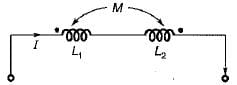When the effect of mutual inductance is subtractive then, we get minimum value of equivalent inductance, Leq = (L1 + L2 - 2M).

Test: Magnetically Coupled Circuits - Question 3

### When two coupled coils of equal self inductance are connected in series in one way, the net inductance is 12 mH and when they are connected in the other way, the net inductance is 4 mH. The maximum value of net inductance when they are connected in parallel In a suitable way is

Detailed Solution for Test: Magnetically Coupled Circuits - Question 3

Given,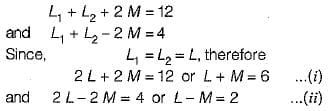From above two equations,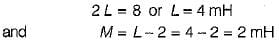To get maximum value in parallel connections,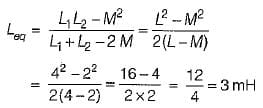Test: Magnetically Coupled Circuits - Question 4

For the ideal transformer shown below, which of the following options is true?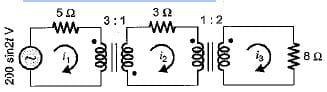Detailed Solution for Test: Magnetically Coupled Circuits - Question 4

8 Ω resistance referred to middle circuit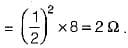Hence, total resistance in the middle circuit
=3 + 2= 5 Ω
This 5 Ω resistance referred to the first circuit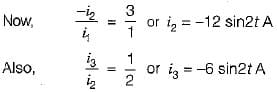Test: Magnetically Coupled Circuits - Question 5

What the greatest mutual inductance that an air- core transformer can have with the selfinductances of 0.3 and 0.7 H respectively?

Detailed Solution for Test: Magnetically Coupled Circuits - Question 5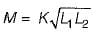For M to be maximum,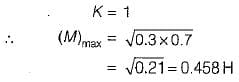Test: Magnetically Coupled Circuits - Question 6

An air-core transformer has primary and secondary currents of i1= 0.2 A and i2 = 0.4 A that produces a flux of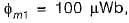,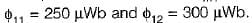. The turns ratio is N1: N2 = 25 : 40. What are the values of L1 and L2 in mH ?

Detailed Solution for Test: Magnetically Coupled Circuits - Question 6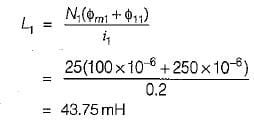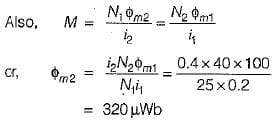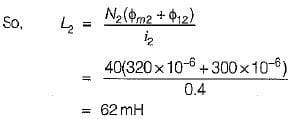Test: Magnetically Coupled Circuits - Question 7

In an ideal transformer with an open-circuited secondary has inductances of L1 = 20 mH, L2 = 32 mH and M = 13 mH. The values of primary and secondary voltages when the primary current is increased at the rate of 0.4 kA/s will be respectively

Detailed Solution for Test: Magnetically Coupled Circuits - Question 7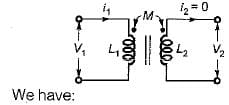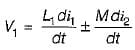(Here, polarity of M is not given in question Hence, it can be +ve or -ve)

and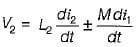Since secondary is open-circuited, therefore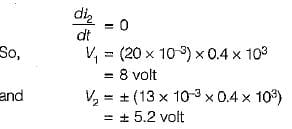Test: Magnetically Coupled Circuits - Question 8

The input inductance of the circuit for ω = 1 rad/s is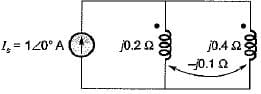Detailed Solution for Test: Magnetically Coupled Circuits - Question 8

Let the voltage drop across the windings be V volt as shown below.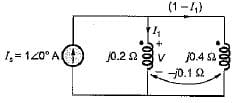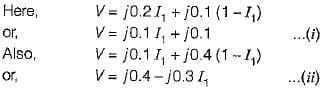(In both the equations above, the effect of mutual inductance is positive since current enters both the dots simultaneously).
On solving equation (i) and (ii), we get

V = j0.175 volt
Let the input inductance be L then,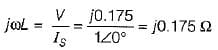∴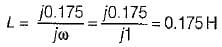Test: Magnetically Coupled Circuits - Question 9

In a certain magnetic circuit, a current of 1 A produces a flux of 1 Wb. if the linear dimensions of the magnetic circuit are doubled, then for producing the same flux, the current would be

Detailed Solution for Test: Magnetically Coupled Circuits - Question 9

We know that,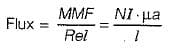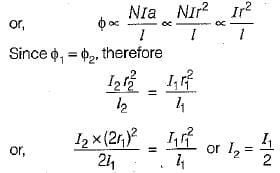Hence , the current will be come half i.e I2 = 1/2 A

Test: Magnetically Coupled Circuits - Question 10

Mutual inductance between two magnetically coupled coils depends on the

## Topicwise Question Bank for Electronics Engineering

101 tests
 Use Code STAYHOME200 and get INR 200 additional OFF Use Coupon Code
Information about Test: Magnetically Coupled Circuits Page
In this test you can find the Exam questions for Test: Magnetically Coupled Circuits solved & explained in the simplest way possible. Besides giving Questions and answers for Test: Magnetically Coupled Circuits, EduRev gives you an ample number of Online tests for practice

101 tests